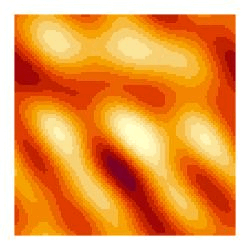# Stefan Siegert

## Animated 2D generative art

After my attempts at static random art, here is a psychedelic animation using generative art.

It uses the random Fourier modes approach, where the Fourier modes change smoothly in time, following a 2nd order autoregressive processes.

Enjoy.

mat0 = mat1 = mat2 = matrix(0, 200, 200)
mat2[1:4, 1:4] = rnorm(16) + 1i * rnorm(16)
while (TRUE) {
mat2[1:4,1:4] = 1.9 * mat1[1:4,1:4] - mat0[1:4,1:4] +
rnorm(16) + 1i * rnorm(16)
image(Re(fft(mat2, inverse=TRUE))); dev.flush(); dev.hold()
mat0 = mat1; mat1 = mat2
}